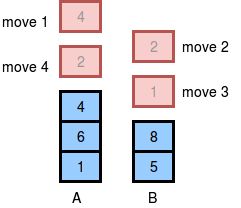## Hackerrank: Game of Two Stacks

Alexa has two stacks of non-negative integers, stack A = [a0, a1, …, an-1]  and stack  B = [b0, b1, …, bm-1] where index 0 denotes the top of the stack. Alexa challenges Nick to play the following game:

• In each move, Nick can remove one integer from the top of either stack  A or stack B.
• Nick keeps a running sum of the integers he removes from the two stacks.
• Nick is disqualified from the game if, at any point, his running sum becomes greater than some integer  given at the beginning of the game.
• Nick’s final score is the total number of integers he has removed from the two stacks.

Given A, B, and x for g games, find the maximum possible score Nick can achieve (i.e., the maximum number of integers he can remove without being disqualified) during each game and print it on a new line.

Input Format

The first line contains an integer, g (the number of games). The 3*g subsequent lines describe each game in the following format:

1. The first line contains three space-separated integers describing the respective values of n (the number of integers in stack A), m  (the number of integers in stack B), and x (the number that the sum of the integers removed from the two stacks cannot exceed).
2. The second line contains n  space-separated integers describing the respective values of a0, a1, …, an-1.
3. The third line contains m space-separated integers describing the respective values of b0, b1, …, bm-1.

Constraints

• 1 <= g <= 50
• 1 <= n,m <= 10^5
• 0 <= ai, bj <= 10^6
• 1 <= x <= 10^9

• 1 <= n,m <= 100 for 50% for  of the maximum score.

Output Format

For each of the g  games, print an integer on a new line denoting the maximum possible score Nick can achieve without being disqualified.

Sample Input 0

1
5 4 10
4 2 4 6 1
2 1 8 5


Sample Output 0

4


Explanation 0

The two stacks initially look like this:The image below depicts the integers Nick should choose to remove from the stacks. We print  as our answer, because that is the maximum number of integers that can be removed from the two stacks without the sum exceeding .(There can be multiple ways to remove the integers from the stack, the image shows just one of them.)

To be honest, I spent an hour on this problem and couldn’t find the optimal solution. Obviously, you can say that there is a brute force solution, but it takes O(2^k), k is the longest steps that can be achieved. Because for each step, we can either go with the top of A or B. Hence, we need two recursive call each steps, or two “branches” each steps. According to Cracking the Coding Interview, time complexity for these problems is O(branches^depth). Replace depth with k, branches with 2.

I was just thinking, to get the longest path, can we just chose the number from either A or B that from that point, we can continue with the furthest distance. Let say we can choose either 5 from A or 6 from B, if we choose 5, we can chop out 5 more elements in A, but if we choose 6 we can chop out 6 elements in B, hence we will choose B. This is short of a greedy solution, and greedy won’t yield optimal result all the time.

So I click on the Editorial part. The editorial doesn’t tell you exactly what is the idea behind the solution, it just says “here is how you solve it”.

The idea is, the longest sequence we can achieve can be formed by either chopping top of A or B continuously until we run out of gas. The format of the final sequence can be:

+ 5 top elements of A, 4 top elements of B
+ 4 top elements of A, 0 top elements of B

The final form is:
+ k1 top elements of A, k2 top elements of B

I think until this point you can probably think of a solution, but if you can’t, let’s continue reading.

To get:
+ 5 top of A, 4 top of B.
We can go from
+ 9 top of A, 0 top of B.
+ 8 top of A, 1 top of B.
….
+ 5 top of A, 4 top of B.

You got the idea, right?

This is the solution in Java, it might not be the most elegant solution but it gets the job done.

https://github.com/MrNocTV/Algorithms/blob/master/SolvedProblems/HackerRank/src/GameOfTwoStacks.java